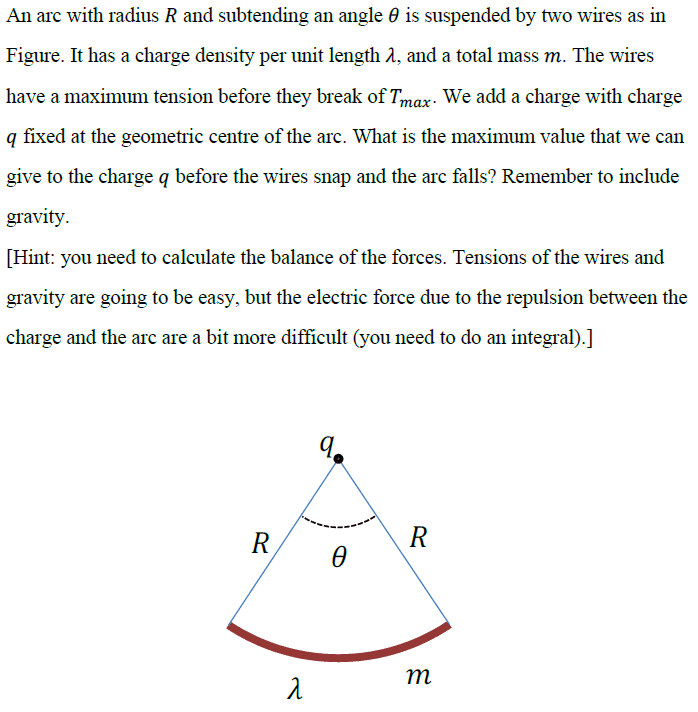# Finding Electric field E due to an arc

• Feeziks
The magnetic field points in the direction of the force, and is given by:$$\vec{B_{z}}=-\frac{1}{2}mG\cos(\theta)$$In summary, the tension in the strings is cancelled out by the weight of the object, and the direction of the force is downwards. The moment the downward force equals the upward force, the strings will snap. The force of repulsion is also downwards, meaning that forces acting downwards are gravity and repulsion, while forces acting upwards are just tension.

#### Feeziks

Homework Statement
An arc with radius 𝑅 and subtending an angle 𝜃 is suspended by two wires as in
Figure. It has a charge density per unit length 𝜆, and a total mass 𝑚. The wires
have a maximum tension before they break of 𝑇max. We add a charge with charge
𝑞 fixed at the geometric centre of the arc. What is the maximum value that we can
give to the charge 𝑞 before the wires snap and the arc falls? Remember to include
gravity.
[Hint: you need to calculate the balance of the forces. Tensions of the wires and
gravity are going to be easy, but the electric force due to the repulsion between the
charge and the arc are a bit more difficult (you need to do an integral).]
Relevant Equations
E = kq/R^2, Weight = mgI need to account for tension, weight, and repulsion.
For the tension, I can draw the x and y component of Tmax and see that the x components of the 2 tensions Tmax will cancel out, and there are 2 y components of the Tmax to factor in.
Weight is just F = mg, where g is acceleration due to gravity.

The direction of Fgravity = downwards while Ftension = upwards. The moment Fupwards =/= Fdownwards, the strings will snap. Frepulsion is downwards, so Forces acting downwards are gravity + repulsion, while Forces acting upwards is tension.

$$dE = k* \frac{dq}{R^2}$$

Solving for dE, I get $$dE = k* \frac{𝜆 d𝜃}{R}$$
since we know S = R𝜃

However, I have trouble solving for E, where

$$E = 2 * \int cos(𝜃/2) \, dE$$ from 0 to infinity.

I am unable to solve this part because cos(𝜃/2) does not converge when 𝜃 goes to infinity. May I know where I went wrong?

Feeziks said:
$$dE = k* \frac{dq}{R^2}$$

Solving for dE, I get $$dE = k* \frac{𝜆 d𝜃}{R}$$
since we know S = R𝜃
It seems ok till here, why don't you try to integrate this?
$$dE = \frac{k \lambda \, d\theta}{R}$$

The critical force balance is
$$2T_{max}\cos\frac{\theta}{2}=mg+F_{coulomb}$$
$$F_{coulomb}=\frac{p}{4\pi\epsilon_0}\frac{qQ}{R^2}$$
where
$$Q=\lambda\theta$$.
You need to investigate unknown coefficient p.

EDIT Thanks to ＠Feeziks in @Post #4　Correction $$Q=\lambda R \theta$$

Last edited:
Pouramat said:
It seems ok till here, why don't you try to integrate this?
$$dE = \frac{k \lambda \, d\theta}{R}$$

I tried to substitute dE into the equation when solving for E, but I get stuck due to the limits and the d𝜃.
If I integrate $$dE = \frac{k \lambda \, d\theta}{R}$$, if the limits are from 0 to infinity, I struggle to calculate due to the infinity involved...

anuttarasammyak said:
The critical force balance is
$$2T_{max}\cos\frac{\theta}{2}=mg+F_{coulomb}$$
$$F_{coulomb}=\frac{p}{4\pi\epsilon_0}\frac{qQ}{R^2}$$
where
$$Q=\lambda\theta$$.
You need to investigate unknown coefficient p.

I have a question, why is $$Q = 𝜆*𝜃$$?
Since we know the sector length is S = R𝜃, so the length of the arc = S, and so I think it should be Q = 𝜆S = 𝜆R𝜃

•anuttarasammyak
There's is something to improve yet.

The electric field points upwards and is given by:
$$\vec{E_{z}}=\int_{-\theta/2}^{\theta/2}\frac{k\lambda d\theta}{R^2}\cos(\theta)d\theta$$